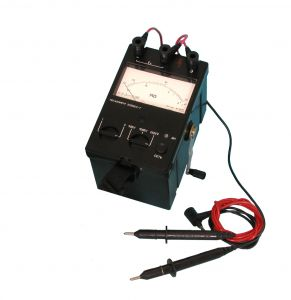`CENNIK PRODUKCJI  ►   PRICE LIST FOR THE PRODUCTION  ►  ПРАЙС ЛИСТ ПРОДУКЦИИ`
ВНИМАНИЕ! С 1 марта 2019 г. изменились цены на трансформаторы тока!

#### CIRCUIT PARAMETERS ZERO-PHASE METER ЦК0220Circuit parameters zero-phase meter ЦК 0220 is designed to measure the strength of short circuit current, electrical impedance, voltage of circuit zero-phase AC networks in 220 V, 50 Hz with earthed neutral.

##### Specifications:

• Measurement range:
• short-circuit current (Іk.z.) in the range of 10 A - 10000 A with an accuracy of ± 5% of the measured value;
• full resistance (Z) in the range of 0,022 Ω - 22 Ω with a tolerance of ± 5% of the measured value;
• the voltage (U) in the range of 180 V - 245 V with a tolerance of ± 1% of measured value in zero-phase circuit and phase-to-ground frequency of 50 Hz, 60 Hz, in which the phase angle between current and voltage (φ) is from 0 to 60 electrical degrees.
• The meter calculates and displays the following circuit parameters:
• active resistance R;
• reactance X;
• the phase angle φ.
• The meter has:
• MCU control and information processing with outputting it to the character display with backlight;
• the memory of the last 10 results of measurements;
• a short-circuit current limiting resistor 0,1 Ω
• The power supply of the meter is performed on the measured network
• The meter automatically disconnects from the power supply with a fall in the external circuit voltage exceeding 36 V, reducing the voltage less than 180 V, or excessing the voltage more than 245 V.
• Operating regime:
• ambient temperature from -10 ° C to + 40 ° C;
• a relative humidity 90% at 30 ° C
• Continuous operation - 8 h.
• The time interval before re-switch is at least 25 min.
• Power consumption (when not MEASUREMENT pressed) of not more than 15 VA.
• Overall dimensions, not more:
• of a meter - 350 mm x 315 mm x 140 mm;
• a bag with a set of connecting wires - 220 mm x 120 mm x 190 mm.
• The weight, not more:
• a meter - 6 kg;
• a meter with a bag and set of connectors - 7 kg.
• Meter ЦК 0220 is designed to replace meters Щ41160 and ЭК0200.• Home
• Theory
• Production
• Certificates
• Contacts
• Questions
• Поверка
• Поддержка
• ### Новости

#### Энергонезависимое питание